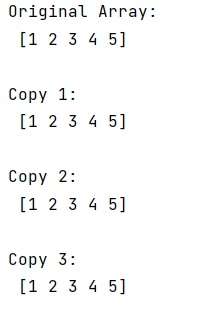# NumPy array assignment with copy

Learn, how assignment with copy of numpy array works?
Submitted by Pranit Sharma, on January 05, 2023

NumPy is an abbreviated form of Numerical Python. It is used for different types of scientific operations in python. Numpy is a vast library in python which is used for almost every kind of scientific or mathematical operation. It is itself an array which is a collection of various methods and functions for processing the arrays.

## Array assignment with copy

There are three common ways to make a copy of a numpy array.

1. A = B: This binds a new name B to the existing object already named A. Then they refer to the same object, so if we modify one in place, we will see the change through the other one too.
2. B[:] = A: This copies the values from A into an existing array B. The two arrays must have the same shape for this to work. B[:] = A[:] does the same thing.
3. copy(A,B): This is not legal syntax. You probably meant B = numpy.copy(A). This is almost the same as 2, but it creates a new array, rather than reusing the B array. If there were no other references to the previous B value, the end result would be the same as 2, but it will use more memory temporarily during the copy.

Let us understand with the help of an example,

## Python code to demonstrate the use of NumPy array assignment with copy

```# Import pandas
import pandas as pd

# Import numpy
import numpy as np

# Creating a numpy array
arr = np.array([1,2,3,4,5])

# Display original array
print("Original Array:\n",arr,"\n")

# Making three copies
# Copy 1
B = arr
print("Copy 1:\n",B,"\n")

# Copy 2
B[:] = arr
print("Copy 2:\n",B,"\n")

# Copy 3
B = np.copy(arr)
print("Copy 3:\n",B,"\n")
```

Output: